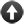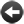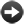§15.5. Arithmetic

We are allowed to perform about the same operations on numbers as are provided by a simple office calculator, starting with addition, subtraction, multiplication and division. We can use the traditional typewriter symbols for these, +, -, * and /, or can spell them out in words as "plus", "minus", "times" (or "multiplied by"), and "divided by". Definitively:

(arithmetic value) + (arithmetic value) ... value

or:

(arithmetic value) plus (arithmetic value) ... value

This phrase performs signed addition on the given values, whose kinds must agree, and produces the result. Examples:

200 + 1 = 201
10:04 AM + two minutes = 10:06 AM

(arithmetic value) - (arithmetic value) ... value

or:

(arithmetic value) minus (arithmetic value) ... value

This phrase performs signed subtraction on the given values, whose kinds must agree, and produces the result. Examples:

200 - 1 = 199
10:04 AM - two minutes = 10:02 AM

(arithmetic value) * (arithmetic value) ... value

or:

(arithmetic value) times (arithmetic value) ... value

or:

(arithmetic value) multiplied by (arithmetic value) ... value

This phrase performs signed multiplication on the given values, whose kinds must be dimensionally compatible, and produces the result. Examples:

201 times 3 = 603
two minutes times 4 = eight minutes

(arithmetic value) / (arithmetic value) ... value

or:

(arithmetic value) divided by (arithmetic value) ... value

This phrase performs signed division on the given values, whose kinds must be dimensionally compatible, and produces the result. Examples:

201 divided by 3 = 67
202 divided by 3 = 67
202.0 divided by 3 = 67.33334
twenty minutes divided by 4 = five minutes
twenty minutes divided by five minutes = 4

Division rounds whole-number values down to the nearest whole number. An attempt to divide a number by 0 will cause a run-time problem message; but an attempt to divide a real number by 0 will instead produce plus infinity or minus infinity.

remainder after dividing (arithmetic value) by (arithmetic value) ... value

This phrase performs signed division on the given values, whose kinds must be dimensionally compatible, and then produces the remainder. Examples:

remainder after dividing 201 by 5 = 1
remainder after dividing twenty minutes by 7 = six minutes

It is mathematically impossible to divide by 0, so any attempt to find the remainder after dividing a number by 0 will cause a run-time problem message. For a real number this won't arise and the remainder will usually be 0.0.

The verbal and symbolic forms of these phrases are equivalent:

the score + 10
the score plus 10

It's probably better style to spell them out in full when writing text, and keep the symbols for writing equations, as we'll see later on in the chapter. (If we do use the symbols, then spaces around them are obligatory: to Inform, they are words which just happen to be spelt with symbols instead of letters.)

Arithmetic often produces fussily exact answers which seem inappropriate in a conversation. Nobody says "Steeple Barton is 7.655 miles down the road", but "Steeple Barton is eight miles down the road" sounds perfectly normal. In order to make that sort of report easier to make, Inform provides another arithmetic operation, one that's not found in most computer programming languages:

(arithmetic value) to the nearest (arithmetic value) ... value

This phrase rounds the given value off, rounding upward in boundary cases. Examples:

201 to the nearest 5 = 200
205 to the nearest 10 = 210
10:27 AM to the nearest five minutes = 10:25 AM

Inform has very few mathematical functions built in as phrases, because these aren't very often needed in story-telling. But it does provide these:

square root of (arithmetic value) ... value

This phrase produces an approximate square root, to the nearest integer, of the given value, which must be of a kind which has square roots. Example:

square root of 16 = 4

Trying to take the square root of a negative number will cause a run-time problem, because then we can't even nearly solve it.

(Warning: this is slow to compute if the Z-machine setting is used. For

best performance, use Glulx.)

real square root of (arithmetic value) ... value

This phrase produces a square root, as accurately as a real number can hold it, of the given value, which must be of a kind which has square roots. Example:

real square root of 2 = 1.41421

The real square root of a negative number is nonexistent.

cube root of (arithmetic value) ... value

This phrase produces an approximate cube root, to the nearest integer, of the given value, which must be of a kind which has cube roots. Example:

cube root of 27 = 3
cube root of -27 = -3

(Warning: this is not very accurate if the Z-machine setting is used. For

best performance, use Glulx.)

We can compare numbers using either the traditional computer-programming symbols, or using words:

if the score is less than 10
if the score < 10

and similarly for "greater than", "at least" and "at most", with the symbols ">", ">=" and "<=". But we are not allowed the equals sign: for that we need only use "is" -

if the score is 10Start of Chapter 15: Numbers and EquationsBack to §15.4. Printing real numbersOnward to §15.6. Powers and logarithms